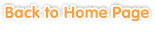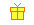Sample questions are shown below. Just click to start!• Chapter 1
Multiplication of Fractions
•
Multiplying Fractions with Whole Numbers••
Multiplying Mixed Numbers with Whole Numbers•
Multiplying Mixed Numbers with Mixed Numbers•
Multiplicative Inverse• Chapter 2
Division of Fractions
•
Dividing Fractions with Whole Numbers••
Dividing Mixed Numbers with Whole Numbers•
Dividing Mixed Numbers with Mixed Numbers• Chapter 3• Chapter 4
Percentages
•
Understanding Percentages•• Chapter 5
Problem Solving:Percentage• Chapter 6
Ratios and Proportions
•
Determining a Ratio•
Solving Proportions• Chapter 7• Chapter 8
Negative Numbers• Chapter 9
Circles
•
Circumference•• Chapter 10
Cylinders and Cones
••
Volume• Chapter 11
Ordered Pairs and the Coordinate Plane
•
Ordered Pairs••
Coordinate Plane and Functions• Chapter 12
Statistics and Probability
•